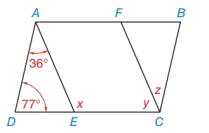Chapter 2.5, Problem 3E### Elementary Geometry for College St...

6th Edition
Daniel C. Alexander + 1 other
ISBN: 9781285195698

#### Solutions

Chapter
Section### Elementary Geometry for College St...

6th Edition
Daniel C. Alexander + 1 other
ISBN: 9781285195698
Textbook Problem
1 views

# Given: A B ¯ ∥ D C ¯ , A D ¯ ∥ B C ¯ , A E ¯ ∥ F C ¯ , with angle measures as indicated Find: x , y and zTo determine

The measure of angles x, y and z.

Explanation

The measure of an exterior angle of a triangle is equal to the sum of the measures of the two nonadjacent interior angles.

Given:

Figure (1)

Definition:

If two parallel lines are cut by a transversal, then the angles on the transversal side are called supplementary and the sum of the measures of the angles is 180°.

Calculate:

mAEC=mD+mDAE(1)

Substitute x for mAEC;77° for mD and 36° for mDAE in the equation (1).

x=77°+36°=113°

Since, AE¯FC¯ and the line CE¯ is traversal then the interior angles are supplementary.

mAEC+mFCE=180°(2)

Substitute 113° for mAEC and y for mFCE in equation (2).

113°+y=180°y=180°113°=67°

Since, AD¯BC¯ and the line CD¯ is traversal then the interior angles are supplementary

### Still sussing out bartleby?

Check out a sample textbook solution.

See a sample solution

#### The Solution to Your Study Problems

Bartleby provides explanations to thousands of textbook problems written by our experts, many with advanced degrees!

Get Started

#### Evaluate the integrals in Problems 1-32. Identify the formula used. 13.

Mathematical Applications for the Management, Life, and Social Sciences

#### Write the sum in expanded form. 3. i=463i

Single Variable Calculus: Early Transcendentals

#### The radius of convergence of is: 1 3 ∞

Study Guide for Stewart's Multivariable Calculus, 8th